# Javascript implementation of Quicksort

Norahiko, a Japanese programmer, wrote an animated demonstration of the sorting algorithm , which is very interesting.

This weekend, I used it as a textbook and studied various sorting algorithms.

Sorting algorithms (Sorting algorithm) is one of the oldest computer science, the basic issue. To become a qualified programmer, you must understand and master various sorting algorithms.

At present, there are about seven or eight kinds of the most common sorting algorithms, among which Quicksort (Quicksort) is the most widely used and faster. It was proposed by the Turing Prize winner CAR Hoare (1934–) in 1960.The idea of ​​”quick sorting” is very simple, the entire sorting process only requires three steps:

(1) In the data set, select an element as the “reference” (pivot).

(2) All elements smaller than “reference” are moved to the left of “reference”; all elements larger than “reference” are moved to the right of “reference”.

(3) Repeat the first and second steps for the two subsets on the left and right of the “benchmark” until there is only one element left in all the subsets.

For example, there is a data set {85, 24, 63, 45, 17, 31, 96, 50}, how to sort it?

In the first step, select the element 45 in the middle as the “benchmark”. (The reference value can be selected arbitrarily, but choosing the middle value is easier to understand.)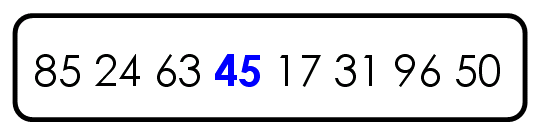The second step is to compare each element with the “benchmark” in order to form two subsets, one “less than 45” and the other “greater than or equal to 45”.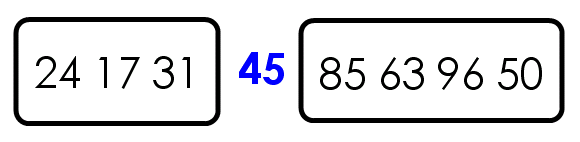In the third step, the first and second steps are repeated for the two subsets until there is only one element left in all the subsets.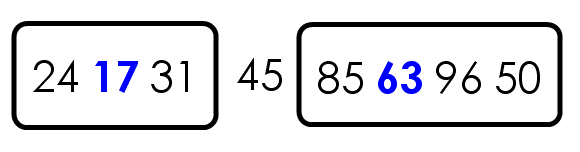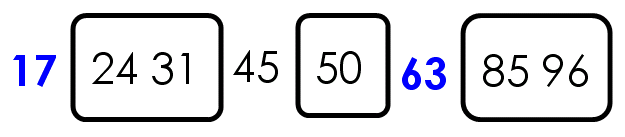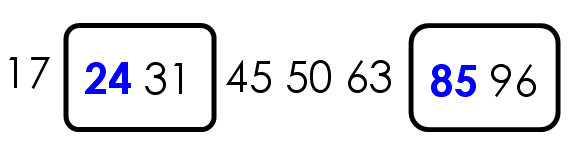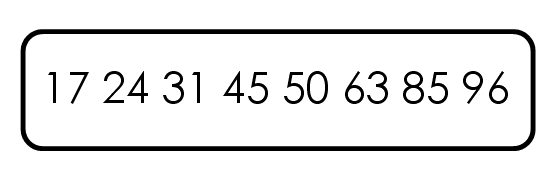Now refer to the information on the Internet ( here and here ) to implement the above algorithm in Javascript language.

First, define a quickSort function whose parameter is an array.

var quickSort = function(arr) {

};

Then, check the number of elements in the array and return if it is less than or equal to 1.

var quickSort = function(arr) {

if (arr.length <= 1) {return arr;}

};

Next, select “Pivot” (pivot), separate it from the original array, and define two empty arrays to store two subsets, one on the left and the other on the right.

var quickSort = function(arr) {

if (arr.length <= 1) {return arr;}

var pivotIndex = Math.floor(arr.length / 2);

var pivot = arr.splice(pivotIndex, 1);

var left = [];

var right = [];

};

Then, start traversing the array, and elements smaller than the “benchmark” are placed in the left subset, and elements larger than the benchmark are placed in the right subset.

var quickSort = function(arr) {

if (arr.length <= 1) {return arr;}

var pivotIndex = Math.floor(arr.length / 2);

var pivot = arr.splice(pivotIndex, 1);

var left = [];

var right = [];

for (var i = 0; i <arr.length; i++){

if (arr[i] <pivot) {

left.push(arr[i]);

} else {

right.push(arr[i]);

}

}

};

Finally, using recursion to repeat this process continuously, you can get the sorted array.

var quickSort = function(arr) {

if (arr.length <= 1) {return arr;}

var pivotIndex = Math.floor(arr.length / 2);

var pivot = arr.splice(pivotIndex, 1);

var left = [];

var right = [];

for (var i = 0; i <arr.length; i++){

if (arr[i] <pivot) {

left.push(arr[i]);

} else {

right.push(arr[i]);

}

}

return quickSort(left).concat([pivot], quickSort(right));

};

When using it, just call quickSort() directly.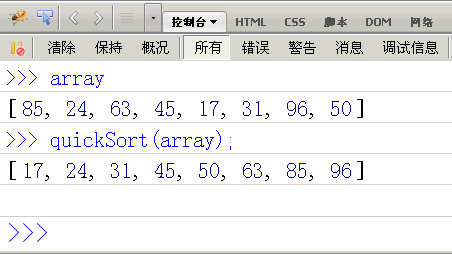(over)